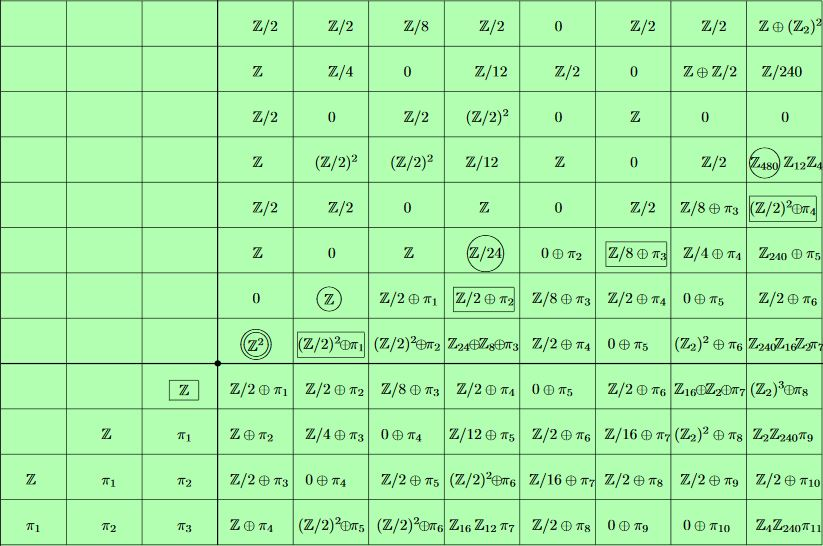nLab equivariant sphere spectrum

Context

Representation theory

representation theory

geometric representation theory

Contents

Idea

Its RO(G)-graded homotopy groups are the equivariant version of the stable homotopy groups of spheres.

Definition

The $G$-equivariant sphere spectrum is the equivariant suspension spectrum of the 0-sphere $S^0 = \ast_+$

$\mathbb{S} = \Sigma^\infty_G S^0$

for $S^0$ regarded as equipped with the (necessarily) trivial $G$-action. It follows that for $V$ an orthogonal linear $G$-representation then in RO(G)-degree $V$ the equivariant sphere spectrum is the corresponding representation sphere $\mathbb{S}(V) \simeq S^V$.

(e.g. Schwede 15, example 2.10)

Properties

Equivariant homotopy groups

Just as for the plain sphere spectrum, the equivariant homotopy groups of the equivariant sphere spectrum in ordinary integer degrees $n$ are all torsion, except at $n = 0$:

$\pi_n^H(\mathbb{S})\otimes \mathbb{Q} = \left\{ \array{ \cdots & for \; n = 0 \\ 0 & otherwise } \right.$

But in some RO(G)-degrees there may appear further non-torsion groups, see the examples below.

In degree 0, the tom Dieck splitting applied to the equivariant suspension spectrum $\mathbb{S} = \Sigma^\infty_G S^0$ gives that $\pi_0^G(\mathbb{S})$ is the free abelian group on the set of conjugacy classes of subgroups of $G$:

(1)$\pi_0^G(\mathbb{S}) \simeq \underset{[H \subset G]}{\oplus} \pi_0^{W_G H}(\Sigma_+^\infty E (W_G H)) \simeq \mathbb{Z}[conjugacy\;classes\;of\;subgroups]$

(e.g. Schwede 15, p. 64)

Examples

$\mathbb{Z}_2$-equivariance

Consider $G= \mathbb{Z}_2$ the cyclic group of order 2 and write $\pi^S_{p,q}$ for the homotopy group in RO(G)-degree given by the representation on $\mathbb{R}^{p+q}$ where $\mathbb{Z}_2$ acts by reflection on the first $p$ coordinates, and trivially on the remaining $q$ coordinates:

The following groups contain $\mathbb{Z}$-summands:

In summary and more generally we have the following $\mathbb{Z}/2$-equivariant stable homotopy groups of spheres in low bidegree:The table shows the $\mathbb{Z}/2$-equivariant stable homotopy groups of spheres $\pi^S_{p,q}$ with $p+q$ increasing horizontally to the right, and $p$ increasing vertically upwards. The origin is the double-circled $\pi^S_{0,0} = \mathbb{Z}^2$. The complex Hopf fibration $\hat\eta$ generates $\pi^S_{1,0} = \mathbb{Z}$, and the quaternionic Hopf fibration generates $\pi^S_{2,1} = \mathbb{Z}/24$

graphics grabbed from Dugger 08, based on Araki-Iriye 82

(beware that Dugger 08 uses a different bi-degree labeling convention: the $(p,q)$ here is $(p+q,p)$ in Dugger 08, matching the coordinates of the above table)

$n_{sgn} + n$$0$$1$$2$$3$
$n_{sgn}$$\pi^{st}_{n_{sgn} + n}$
$2$$0$$0$$\mathbb{Z} = \langle (h_{\mathbb{C}})^2\rangle$$\mathbb{Z}_{24} = \langle h_{\mathbb{H}}\rangle$
$1$$0$$\mathbb{Z} = \langle h_{\mathbb{C}}\rangle$$\pi_1^{st} \oplus \mathbb{Z}_2$$\pi^{st}_2 \oplus \mathbb{Z}_2$
$0$$\pi_0^{st} \oplus \mathbb{Z}$$\pi_1^{st} \oplus (\mathbb{Z}_2)^2$$\pi_2^{st} \oplus (\mathbb{Z}_2)^2$$\pi_3^{st} \oplus \mathbb{Z}_8 \oplus \mathbb{Z}_{24}$

Cyclic ($G_D \hookrightarrow SO(2)$-)equivariance

The global equivariant sphere spectrum for all the cyclic groups over the circle group is canonically a cyclotomic spectrum and as such is the tensor unit in the monoidal (infinity,1)-category of cyclotomic spectra (see there).

General

General lecture notes include

Discussion in rational equivariant stable homotopy theory includes

The sphere spectrum in global equivariant homotopy theory is discussed in

Relation to equivariant framed bordism

• Czes Kosniowski, Equivariant Stable Homotopy and Framed Bordism, Transactions of the American Mathematical Society Vol. 219 (1976), pp. 225-234 (jstor:1997591)

$\mathbb{Z}/2$-equivariance

Discussion of $G$-equivariant homotopy groups for $G = \mathbb{Z}/2$ is in

• Peter Landweber, On Equivariant Maps Between Spheres with Involutions, Annals of Mathematics Second Series, Vol. 89, No. 1 (Jan., 1969), pp. 125-137 (jstor)

• Shôrô Araki, Kouyemon Iriye, Equivariant stable homotopy groups of spheres with involutions. I, Osaka J. Math. Volume 19, Number 1 (1982), 1-55. (Euclid:1200774828)

• Kouyemon Iriye, Equivariant stable homotopy groups of spheres with involutions. II, Osaka J. Math. Volume 19, Number 4 (1982), 733-743 (euclid:1200775536)

• Daniel Dugger, Daniel Isaksen, $\mathbb{Z}/2$-equivariant and R-motivic stable stems, Proceedings of the American Mathematical Society 145.8 (2017): 3617-3627 (arXiv:1603.09305)

with exposition in

$\mathbb{Z}/4$-equivariance

Discussion for $G = \mathbb{Z}/4$ is in

• M. C. Crabb, Periodicty in $\mathbb{Z}/4$ equivariant stable homotopy theory, in Mark Mahowald and Stewart Priddy (eds.) Algebraic Topology, Proceedings of the International Conference held March 21-24 (1988) Contemporary Mathematics, volume 96, 1989 (pdf, publisher page)

General background includes